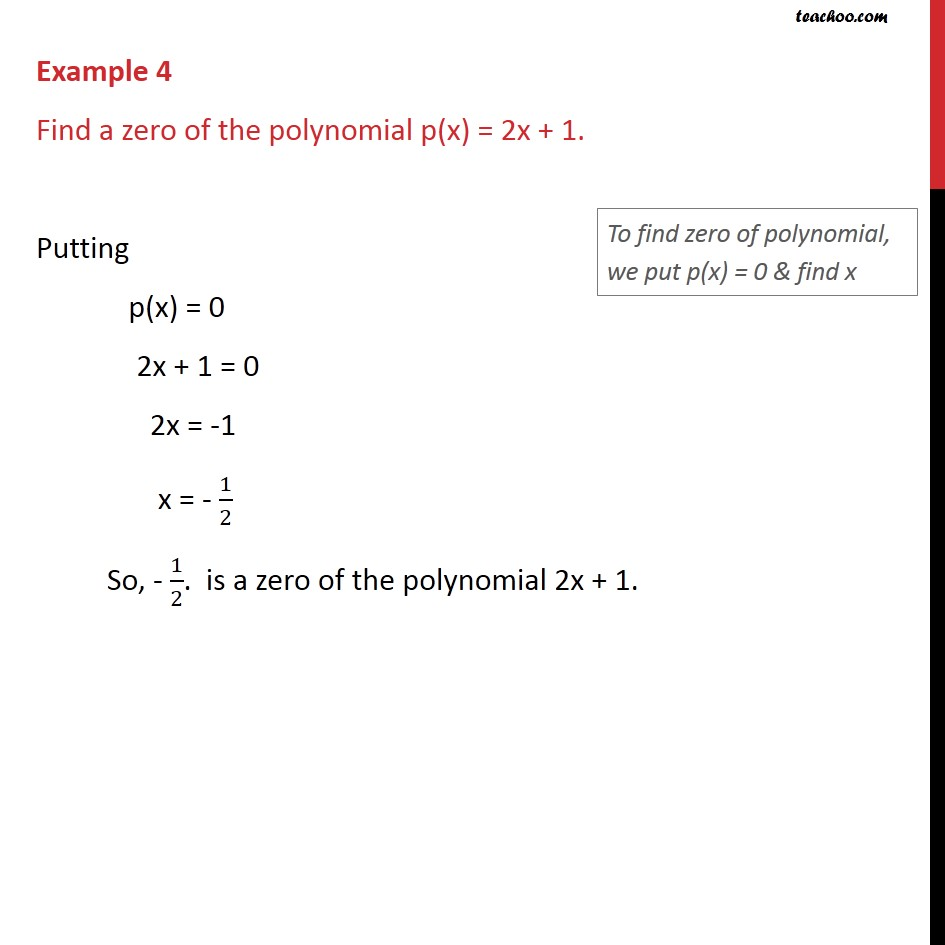1. Chapter 2 Class 9 Polynomials (Term 2)
2. Concept wise
3. Finding Zeroes of a polynomial

Transcript

Example 4 Find a zero of the polynomial p(x) = 2x + 1. Putting p(x) = 0 2x + 1 = 0 2x = -1 x = - 1/2 So, - 1/2. is a zero of the polynomial 2x + 1.